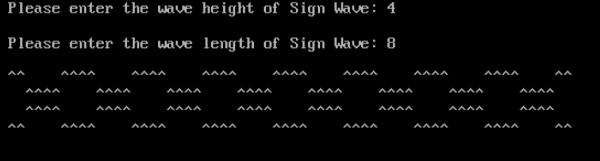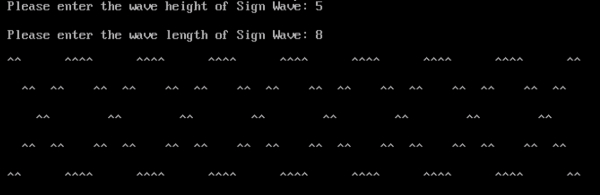# Print the Mirror Image of Sine-Wave Pattern in C

## Program Description

A sine wave or sinusoid is a mathematical curve that describes a smooth periodic oscillation. A sine wave is a continuous wave. It is named after the function sine, of which it is the graph. It occurs often in pure and applied mathematics, as well as physics, engineering, signal processing and many other fields.

Print the Mirror Image of Sine-Wave Pattern based on the Wave Height and Wave Length

## Algorithm

Accept the Wave Height and Wave Length

Print the Wave Sign for the Wave Height and the Wave Length.

## Example

/* Program to print the mirror image of Sine Wave*/
#include<stdio.h>
int main(){
int wave_height;
int wave_length;
int i,j,k;
clrscr(); /*Clears the Screen*/
printf("Please enter the wave height of Sign Wave: ");
scanf("%d", &wave_height);
printf("");
printf("Please enter the wave length of Sign Wave: ");
scanf("%d", &wave_length);
printf("");
for(i = 1; i <= wave_height; i++) {
for(j = 1; j <= wave_length; j++){
for(k = 1; k <= wave_height; k++) {
if(i == k || i + k == wave_height + 1) {
printf("^^");
}
else {
printf(" "" ");
}
}
}
printf("");
}
getch();
return 0;
}

## Output